# A Sine Signal

 Tip When the training set is not similar to the validation set, an ANN will always produce unexpected results. In the problem of the last section the ANN was trained using perfect sine waves at its input and its output. However, the validation set included noisy signals that were never used for training;as a result the network did not know how to handle the noisy input.

 Problem 1 Create a New Project called SineSignal to build an appropriate training set for learning of the sine wavez = sin(x) using a ANN for auto-association. Use 2048 training cases and 64 inputs. The input training set must include noisy sine waves and the target must include clean sine waves as shown in the figure below. The training set must include the same phasein eight different training cases.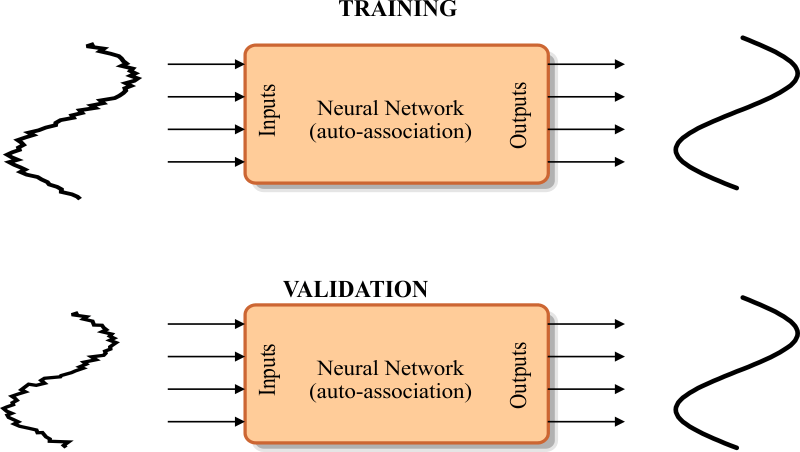Solution 1 After editing the BuildTrainSet.lab file,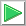Run click the button to execute the code. If you do not have any errors, the training set will be generated and displayed on the variable list and the file list. Click on the file to see its contents.The training set input is a noisy sine signal; the training set target is a clean sine signal of the same phase as the input.

 SineSignal\BuildTrainSet.lab int numCases = 2048/8; int numInputs = 64; double range = 2.0*3.1415926; double deltaInput = range/numInputs; double deltaCase = range/numCases; //________________________________ Create the target Matrix trainSetTarget; trainSetTarget.Create(numCases, numInputs); int i; int j; double phase = 0.0; for(i=0; i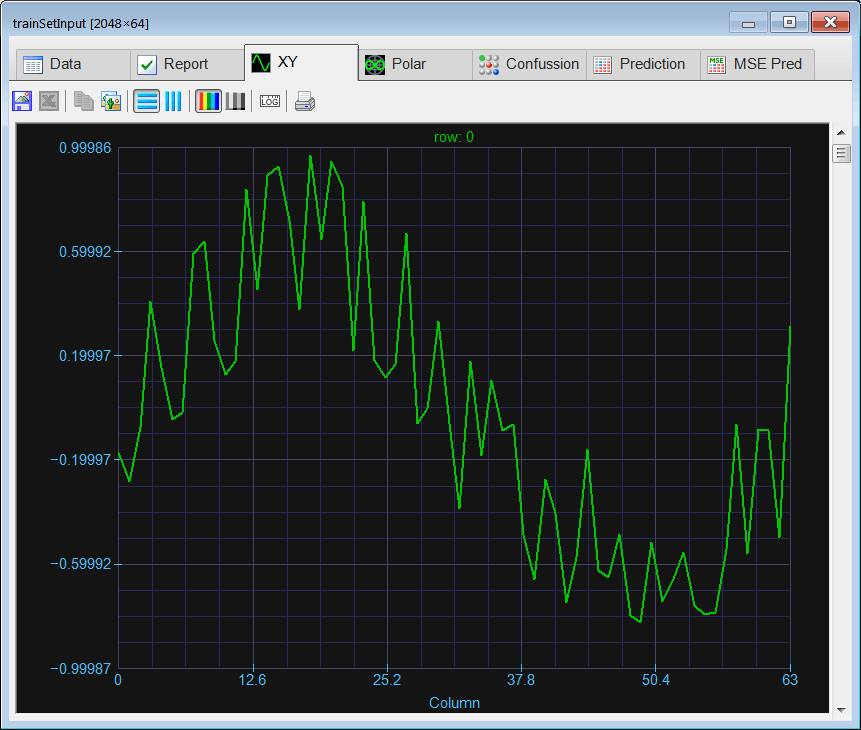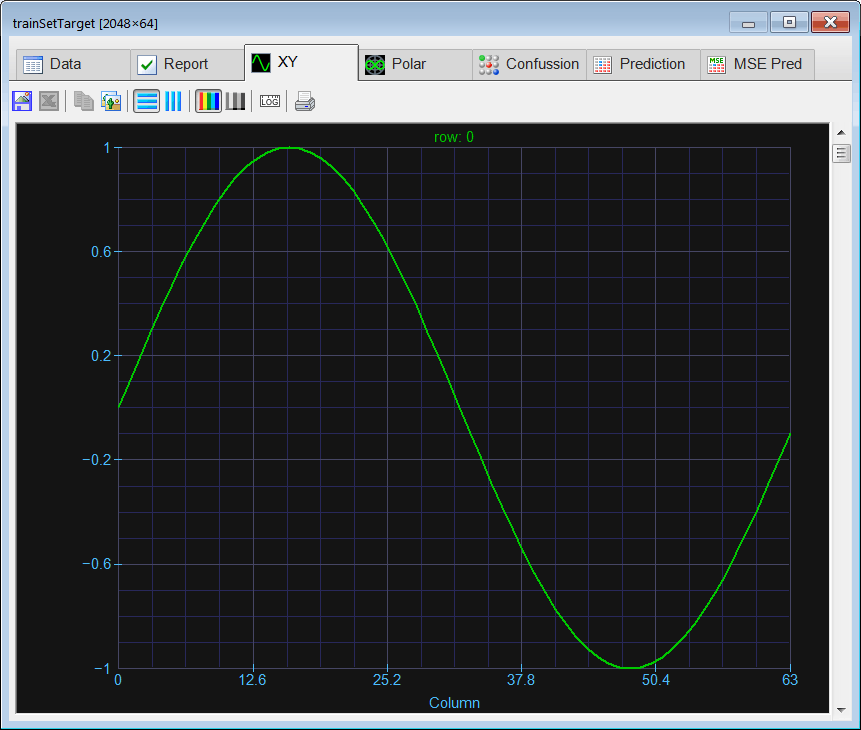Problem 2 (a) In last problem, how many rows did the training set input have before calling the function AppendDown? (b) How many rows did the training set input have after the first call to AppendDown? (c) How many rows did the training set have after the second call to AppendDown?

 Problem 3 Edit theBuilValidSet.lab file to buildthe validation set using the training set. Contaminate the target training set with 10% of noise to create the input validation set. The target of the validation set is the same as the target of the training set.

 Solution 3 After editing the file,Run click the button to execute the code. If you do not have any errors, the validation set will be generated and displayed on the variable list and the file list. Use the scroll bar at the right to visualize each validation case. Each case should be a noisy sine wave at a different phase. Observe that in this specific case the validation set input and target are the same.LABCOSineSignal\BuildValidSet

 Problem 4 Edit the Train.lab file to design and train an ANN for the sine wave. Use one hidden layer and 14 neurons in this layer. Train the ANN using simulated annealing and the conjugate gradient method. Because of the number of outputs, it is not possible to use the method ofLevenberg Marquardt.

 Solution 4 After editing the file,Run click the button to execute the code. If you do not have any errors, the ANN will be trained when the code execution stops. Double click the network in the variable list to see the network,, select the trainSetInput.csv file for input, and select trainSetTarget.csv file for target. You will be able to review the network behavior in real time by moving the scrollbar at the right.

 SineSignal\Train.lab int numInputs = 64; int i; //_________________________ Network Setup LayerNet net; net.Create(numInputs, 14, 0, numInputs); for(i = 0; i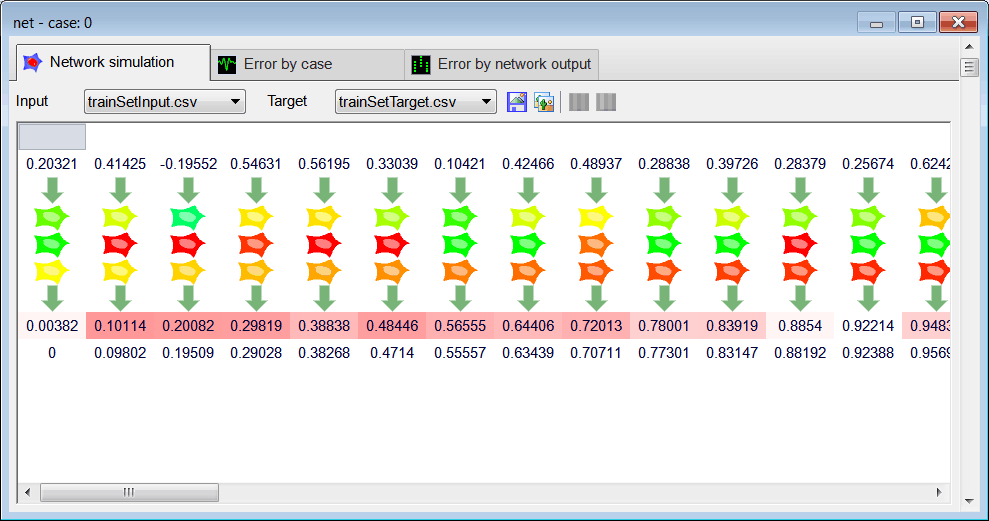Problem 5 Edit the CheckTraining.lab file to check the training: (a) Compute the mean squared error for the ANN using the training set. (b) Plot the error for each training case. (c) Save the plot as a vector image (checkTraining.pdf and checkTraining.emf)

 Solution 5 (a) After editing the file,Run click the button to execute the code. If you do not have any errors, the mse will be displayed in the variable list. Click the variable called output to review the network behavior. If you network was trained properly, you should be able to see that the output of the network is a perfect sine wave for all of training cases (use the scroll bar at the right to verify the output for other training cases).

 SineSignal\CheckTraining.lab //_________________________________________ Load the training set Matrix trainSetInput; trainSetInput.Load(); Matrix trainSetTarget; trainSetTarget.Load(); //_________________________________________ Load the ANN LayerNet net; net.Load(); //_________________________________________ Run Matrix output = net.Run(trainSetInput); double mse = ComputeMse(output, trainSetTarget); //_________________________________________ Relative Error Vector error = ComputeRelError(output, trainSetTarget); XyChart checkTraining; checkTraining.SetCaption("case", false, "Relative Error", false); checkTraining.AddGraphY(error, "Relative Error", 2, 1, 0, 255, 0); checkTraining.SetLogScale(false, true); checkTraining.SetLimits(0, trainSetTarget.GetRowCount(), 1.0e-5, 1.0); checkTraining.SetColorMode(2); checkTraining.SaveAndShow(); checkTraining.SavePDF(0.0); checkTraining.SaveEMF();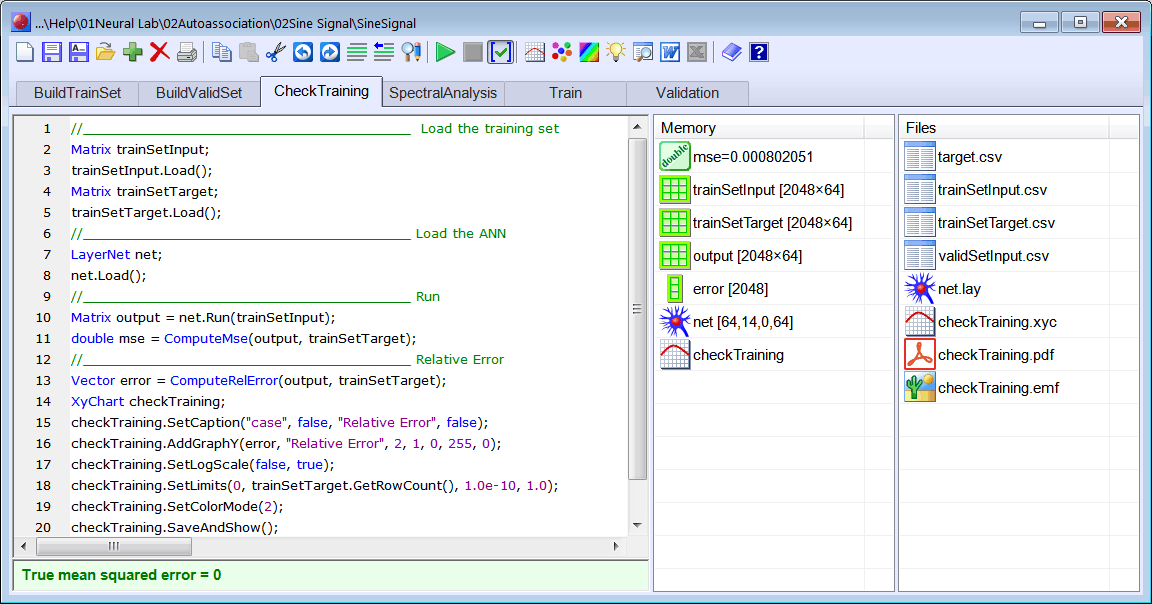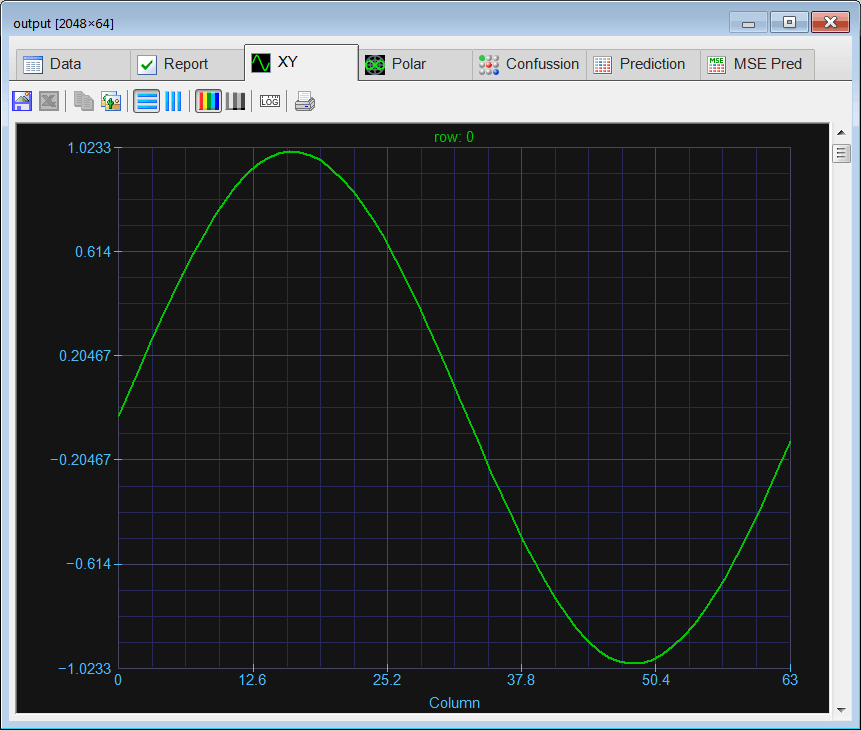Problem 6 Edit the Validation.lab file to perform the validation of the ANN. (a) Compute the mean squared error for the ANN using the validation set. (b) Plot the error for each validation case. (c) Save the plot as a vector image (validation.pdf and validation.emf)

 Solution 6 (a) After editing the file,Run click the button to execute the code. . If you do not have any errors, the mse will be displayed in the variable list. As the value of the msefor both the training set and the validation is somehowsimilar, the network was properly designed and trained. Click the variable called output to review the network behavior, observe how the network is able to considerably eliminate the noise of the input signal for each training case.

 SineSignal\Validation.lab //_________________________________________ Load the validation set Matrix validSetInput; validSetInput.Load(); Matrix trainSetTarget; trainSetTarget.Load(); //_________________________________________ Load the ANN LayerNet net; net.Load(); //_________________________________________ Run Matrix output = net.Run(validSetInput); //_________________________________________ Compute the mean squared error double mse = ComputeMse(output, trainSetTarget); //_________________________________________ Relative Error Vector error = ComputeRelError(output, trainSetTarget); XyChart validation; validation.SetCaption("case", false, "Relative Error", false); validation.AddGraphY(error, "Relative Error", 2, 1, 0, 255, 0); validation.SetLogScale(false, true); validation.SetLimits(0, trainSetTarget.GetRowCount(), 1.0e-5, 1.0); validation.SetColorMode(2); validation.SaveAndShow(); validation.SavePDF(0.0); validation.SaveEMF(); Vector target0 = trainSetTarget.GetRowVec(0); Vector target199 = trainSetTarget.GetRowVec(199); Vector target399 = trainSetTarget.GetRowVec(399); // Vector output0 = output.GetRowVec(0); Vector output199 = output.GetRowVec(199); Vector output399 = output.GetRowVec(399); // Vector input0 = validSetInput.GetRowVec(0); Vector input199 = validSetInput.GetRowVec(199); Vector input399 = validSetInput.GetRowVec(399);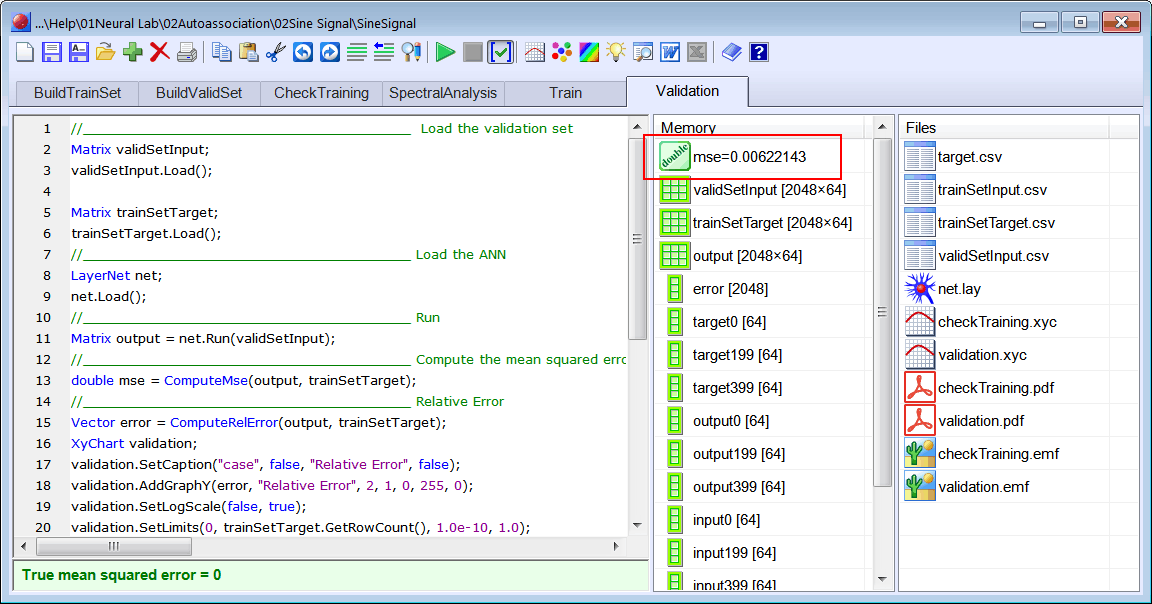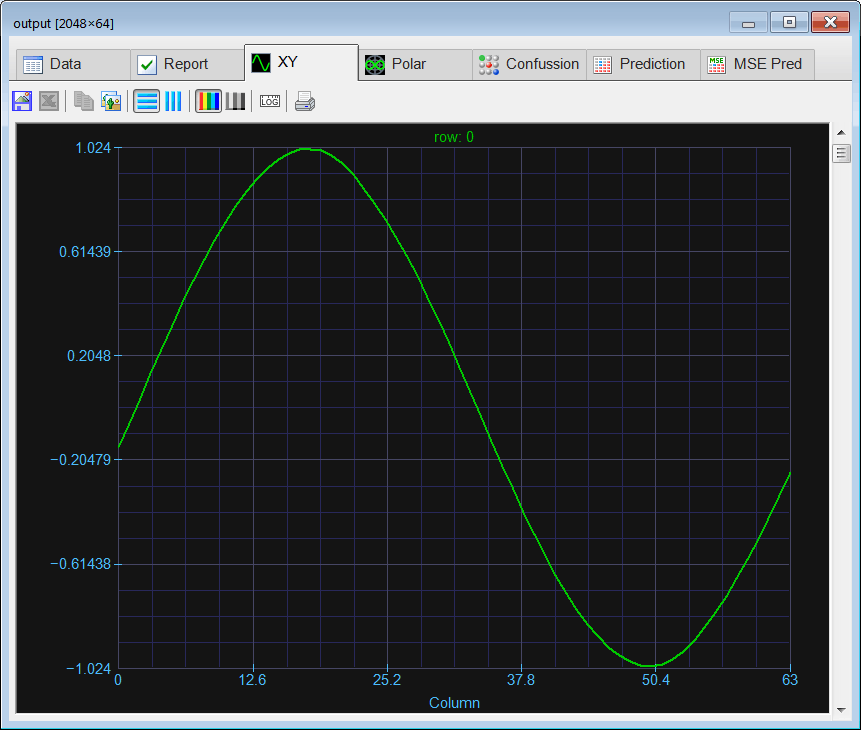Problem 7 Repeat Problem 1 to 6 using: (a) signals contaminated with 40% of noise, (b) signals contaminated with 80% of noise.

 Problem 8 Generate a report in Microsoft Word. Write some conclusions in the report focusing on the problems that were faced during the simulation and how these problems were or could be solved.

 Problem 9 A student found a database using the Internet. The database was composed of 200 training cases. To be able to use more neurons in the hidden layer (and thus reduce the MSE for training), the student duplicated the 200 training cases to build a database with 400 cases. Is her approach correct?

 Problem 10 Explain why in problem 1 we have used the same cases eight times.Explique porqué en el problema 1 nosotros hemos usado ochos veces los mismos casos.

 Problem 11 Repeat Problems 1 to 6 using Matlab.Create a folder called SineSignalMatlabCopy the previously CSV files: trainSetInput.csv, validSetInput.csv, trainSetTarget.csvCreate and execute the file Trainx.m (Try several training methods: traingdx, trainbfg, trainlm, …)Use the csvread command to load the CSV file in Matlab, Transpose the matrixes using an apostrophe, i.e., A = B';Create and execute the file CheckTraining.mCreate and execute the file Validation.mCreate and execute the file Simulatex.m